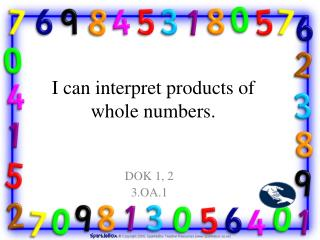DownloadDownload PresentationI can interpret products of whole numbers.

# I can interpret products of whole numbers.

Download Presentation## I can interpret products of whole numbers.

- - - - - - - - - - - - - - - - - - - - - - - - - - - E N D - - - - - - - - - - - - - - - - - - - - - - - - - - -
##### Presentation Transcript

1. I can interpret products of whole numbers. DOK 1, 2 3.OA.1

2. I can model multiplication facts by forming groups to represent the factors. DOK 1, 2 3.OA.1

3. I can interpret quotients of whole numbers. DOK 1, 2 3.OA.2

4. I can model division facts by separating objects into equal shares. DOK 1, 2 3.OA.2

5. I can solve multiplication word problems by using equations. (within 100) DOK 1, 2 3.OA.3

6. I can solve division word problems by using equations. (within 100) DOK 1, 2 3.OA.3

7. I can solve multiplication word problems by using drawings. (within 100) DOK 1, 2 3.OA.3

8. I can solve division word problems by using drawings. (within 100) DOK 1, 2 3.OA.3

9. I can determine the unknown whole number in a multiplication problem. (missing value) DOK 1, 2 3.OA.4

10. I can determine the unknown whole number in a division problem. DOK 1, 2 3.OA.4

11. I can apply the commutative property to solve multiplication problems. DOK 1, 2 3.OA.5

12. I can apply turn-around facts. DOK 1, 2 3.OA.5

13. I can apply associative property to solve multiplication problems. DOK 1, 2 3.OA.5

14. I can choose two numbers to easily multiply then multiply that product by the remaining number. DOK 1, 2 3.OA.5

15. I can apply distributive property to solve multiplication problems DOK 1, 2 3.OA.5

16. I can change my problem to make it easier to multiply. DOK 1, 2 3.OA.5

17. I can use multiplication fact families to solve division problems. DOK 1, 2 3.OA.6

18. I can recall, from memory, all products of two one-digit numbers. DOK 1, 2 3.OA.7

19. I can fluently multiply using properties and strategies. DOK 1, 2 3.OA.7

20. I can fluently divide using properties and strategies. DOK 1, 2 3.OA.7

21. I can solve two-step word problems using addition. DOK 1, 2, 3 3.OA.8

22. I can solve two-step word problems using subtraction. DOK 1, 2, 3 3.OA.8

23. I can solve two-step word problems using multiplication. DOK 1, 2, 3 3.OA.8

24. I can solve two-step word problems using division. DOK 1, 2, 3 3.OA.8

25. I can represent addition word problems using equations. DOK 1, 2, 3 3.OA.8

26. I can represent subtraction word problems using equations. DOK 1, 2, 3 3.OA.8

27. I can represent multiplication word problems using equations. DOK 1, 2, 3 3.OA.8

28. I can represent division word problems using equations. DOK 1, 2, 3 3.OA.8

29. I can use mental computation strategies to see if my answer is reasonable. DOK 1, 2, 3 3.OA.8

30. I can use mental estimation strategies to see if my answer is reasonable. DOK 1, 2, 3 3.OA.8

31. I can identify arithmetic patterns in addition tables. DOK 1, 2, 3 3.OA.9

32. I can identify arithmetic patterns in multiplication tables. DOK 1, 2, 3 3.OA.9

33. I can explain the arithmetic patterns in addition. DOK 1, 2, 3 3.OA.9

34. I can explain the arithmetic patterns in multiplication. DOK 1, 2, 3 3.OA.9

35. I can use place value to round whole numbers to the nearest 10. DOK 1 3.NBT.1

36. I can use place value to round whole numbers to the nearest 100. DOK 1 3.NBT.1

37. I can fluently add numbers up to 1,000. DOK 1, 2 3.NBT.2

38. I can fluently subtract numbers up to 1,000. DOK 1, 2 3.NBT.2

39. I can multiply one-digit whole numbers by multiples of 10. (10-90) DOK 1, 2 3.NBT.3

40. I can define fractions as parts of a whole. DOK 1, 2 3.NF.1

41. I can determine the individual parts within a fraction. (numerator) DOK 1, 2 3.NF.1

42. I can determine the number of equal parts within a fraction. (denominator) DOK 1, 2 3.NF.1 DOK 1, 2 3.NF.2

43. I can identify a fraction on a number line. DOK 1, 2 3.NF.2a

44. I can identify a fractional part on a number line. DOK 1, 2 3.NF.2b

45. I can represent a fraction on a number line when 1 is the numerator. (Ex. Using a number line 0-1 to show each section marked between 0-1 represents 1/n .) DOK 1, 2 3.NF.2b

46. I can represent a fraction on a number line when the numerator is more than 1. (Ex. Using a number line 0-1 to show each section marked increases the numerator.) DOK 1, 2 3.NF.2b

47. I can generate simple, equivalent fractions. DOK 1, 2 3.NF.2b DOK 1, 2, 3 3.NF.3

48. I can recognize when two fractions are equivalent when they are the same size or the same point on a number line. DOK 1, 2, 3 3.NF.3a

49. I can recognize simple equivalent fractions. DOK 1, 2, 3 3.NF.3b